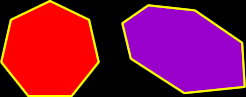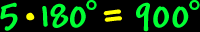# Polygons - Heptagons

Properties of heptagons, interior angles of heptagons

Polygons:  Properties of HeptagonsSum of the Interior Angles of a Heptagon:
 This image shows the process for a HEXAGON:Using the same methods as for hexagons to the right (I'll let you do the pictures)... To find the sum of the interior angles of a heptagon, divide it up into triangles... There are five triangles...  Because the sum of the angles of each triangle is 180 degrees...  We getSo, the sum of the interior angles of a heptagon is 900 degrees.Regular Heptagons:
The properties of regular heptagons:All sides are the same length (congruent) and all interior angles are the same size (congruent).

To find the measure of the interior angles, we know that the sum of all the angles is 900 degrees (from above)...  And there are seven angles...So, the measure of the interior angle of a regular heptagon is about 128.57 degrees.The measure of the central angles of a regular heptagon:To find the measure of the central angle of a regular heptagon, make a circle in the middle...  A circle is 360 degrees around...  Divide that by seven angles...So, the measure of the central angle of a regular heptagon is about 51.43 degrees.